Latest Banking jobs   »

# Quantitative Aptitude Quiz For IRDA AM 2023 -19th May

Directions (1-5): The following table shows the total no. students and percentage of students present on a particular day out of them for two schools A and B in different classes.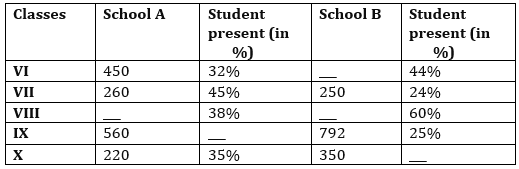Q1. If total number of students in class VIII in both the school together are 1625, while total students present in both the classes together are 766, then find difference between number of students in class VIII in both the schools?
(a)250
(b) 275
(c) 225
(d) 300
(e) None of these

Q2. The number of students present in class VI in school A are approximately what percent of number of students present in class IX in school B?
(a)65%
(b) 70%
(c) 75%
(d) 73%
(e) 80%

Q3. If number of students present in class VI in school B are 2 less than double of the number of students present in same class in school A, then find the total number of students in class VI in school B.
(a)625
(b) 650
(c) 600
(d) 700
(e) None of these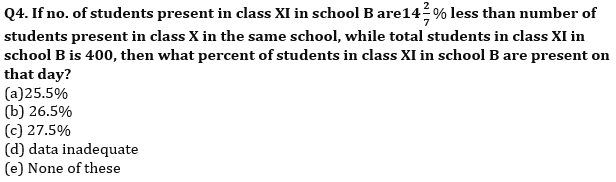Q5. Find the total number of students present in class VII in both the schools together?
(a) 157
(b) 187
(c) 167
(d) 177
(e) None of these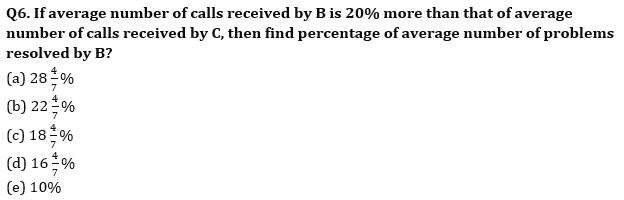Directions (6 -10): Table given below shows average number of calls received (related sim connection problem) by six employees and average number of problems resolved by these six employees. Some data is missing, Read the data carefully and answer the questions.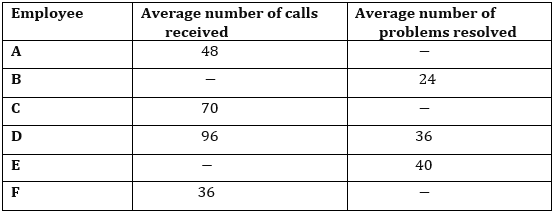Q7. Average number of calls received by E is 10% more than that of received by B. If average number of calls received by E is eight less than that of average number of calls received by D, then find average number of problems which B did not resolve?
(a) 24
(b) 32
(c) 36
(d) 44
(e) 56

Q8. If percentage of average number of problems resolved by A is 41⅔%, then find average number of problems which A did not resolve are what percent of average number of calls received by C?
(a) 35%
(b) 44%
(c) 48%
(d) 40%
(e) 50%

Q9. If average number of calls received by E is 24 more than average of average number of calls received by A & D, then find ratio of average number of problems which E did not resolve to average number of problems which D did not resolve?
(a) 14 : 17
(b) 14 :1 5
(c) 13 : 15
(d) 3 : 5
(e) 4 : 5

Q10. Average number of calls received by E is 37.5% more than that of received by A, while average number of calls received by B is 20% more than that of received by C. If F resolved 50% of average number of calls received by him, then find average number of problems resolved by F is what percent of total average number of calls received by all six?
(a) 3.5%
(b) 4.5%
(c) 2.5%
(d) 1.5%
(e) 5%

Solutions: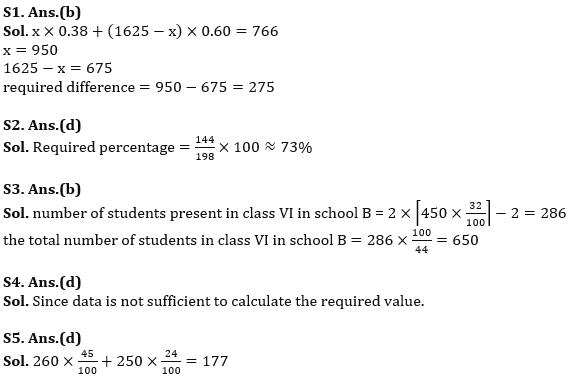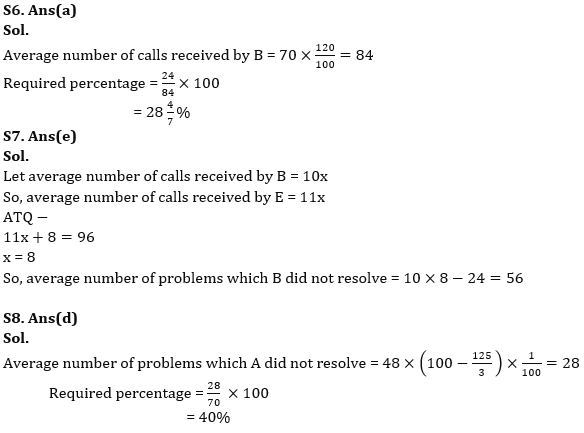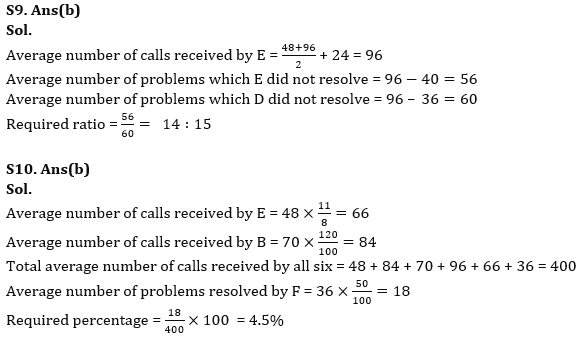## FAQs

### How many sections are there in the IRDAI exam?

There are 4 sections in the IRDAI assistant manager online preliminary exam. They are Reasoning, English Language, General awareness, Quantitative aptitude.

#### Congratulations!Union Budget 2023-24: Free PDF